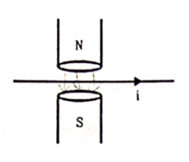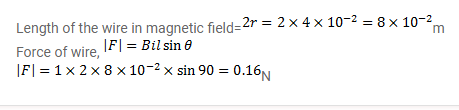# A magnetic field of strength 1.0T is produced by a strong electromagnet

Question:

A magnetic field of strength $1.0 \mathrm{~T}$ is produced by a strong electromagnet in a cylindrical region of radius $4.0 \mathrm{~cm}$ as shown in figure. A wire, carrying a current of $2.0 \mathrm{~A}$, is placed perpendicular to and intersecting the axis of the cylindrical region. Find the magnitude of the force acting on the wire.Solution: